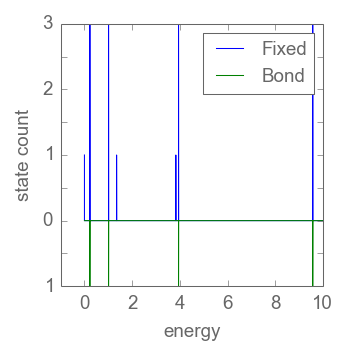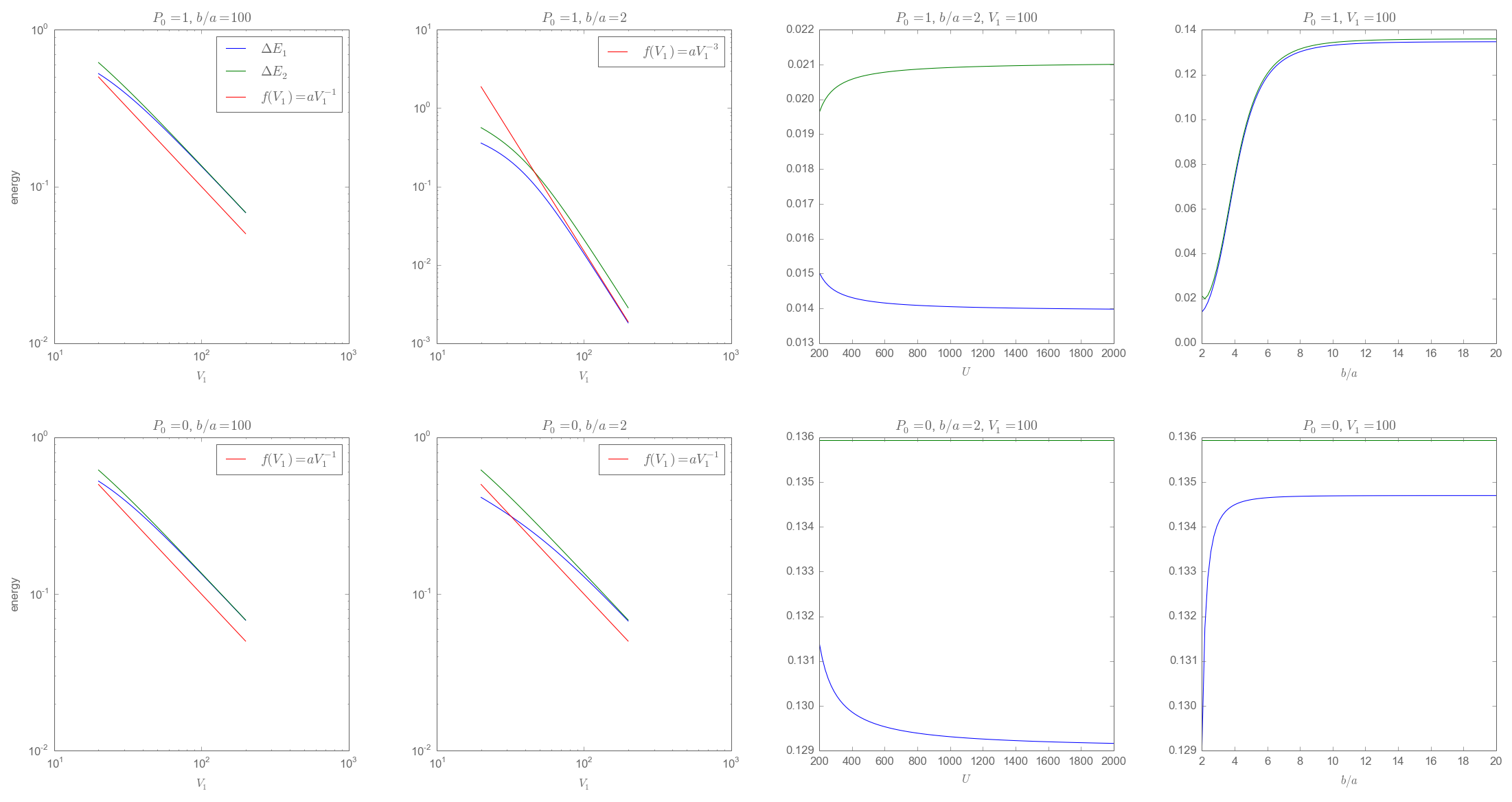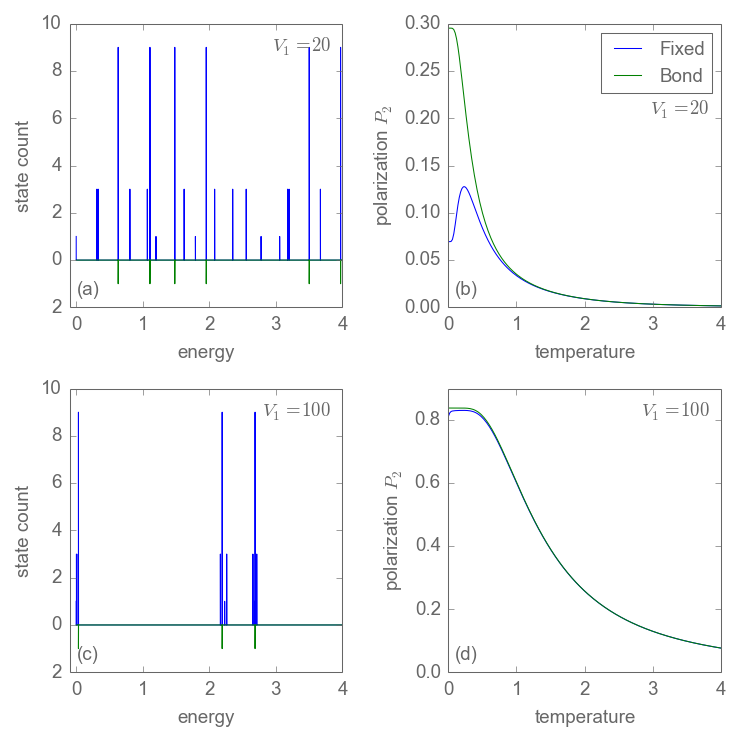# Bond approximation¶

In this notebook I'll have a look at how exactly the bond approximation works. Starting point is the fixed charge system, with two electrons per cell and therefore 28 states per cell. We can represent these 28 states in a different basis, the bond basis: Two electrons make up a bond and there are six bonds, four on the edges of the cell and two on the diagonal. Each bond consists of three triplet and and singlet state. Together with the four double occupied states, this gives the 28 states ($6 \cdot4 + 4 = 28$).

Now we neglect all doubly occupied states and the spin, reducing the four states for every bond to only one, yielding six states (the six bonds) per cell in total. In what limits is this approximation valid and how does it actually work?

One obvious requirement is that $T \ll U$ (in my code I usually use $V0 = V_0 = U$), the doubly occupied states need to be gapped out.

By representing each bond by only one state we assume that the triplet and singlet states are equivalent and energetically degenerate. This is not correct. In a simplified picture we would expect that virtual excitations to higher energy states yield a lower enegy for the singlet state, similar in fashion to how the Heisenberg model can be derived as the low energy limit of the Hubbard model (where we have $J \sim \frac{t^2}{U}$).

We plot the (low) energy histogram of both the fixed charge and the bond model for a single active QCA cell (there's still the static driving cell) and suitably chosen parameters.

In :
# increases the resolution of the pngs displayed inline,
# so they are big enough even for a small figsize
# I should also be able to set this in ipython_notebook_config.py
# in the profile directory
%matplotlib inline
c = %config InlineBackend.rc
c['savefig.dpi'] = 150
%config InlineBackend.figure_format='png'
%config InlineBackend.rc = c
%matplotlib inline

# imports
import matplotlib.pyplot as plt
import matplotlib as mpl
import numpy as np
import qca
print(qca.__version__)

8.2.0

In :
grey = '#666666'
lw = 0.5
margin = 0.15
mpl.rcParams['figure.figsize'] = (5,5)
mpl.rcParams['font.size'] = 9
mpl.rcParams['font.sans-serif'].insert(0,'Open Sans')
mpl.rcParams['legend.fontsize'] = 'medium'
mpl.rcParams['lines.linewidth'] = lw
mpl.rcParams['patch.linewidth'] = lw
mpl.rcParams['axes.linewidth'] = lw
mpl.rcParams['patch.edgecolor'] = grey
mpl.rcParams['axes.edgecolor'] = grey
mpl.rcParams['axes.labelcolor'] = grey
mpl.rcParams['text.color'] = grey
mpl.rcParams['xtick.color'] = grey
mpl.rcParams['ytick.color'] = grey
mpl.rcParams['xtick.major.size'] = 3
mpl.rcParams['xtick.minor.size'] = 1
mpl.rcParams['xtick.major.width'] = 0.3
mpl.rcParams['xtick.minor.width'] = 0.3
mpl.rcParams['ytick.major.size'] = 3
mpl.rcParams['ytick.minor.size'] = 1
mpl.rcParams['ytick.major.width'] = 0.3
mpl.rcParams['ytick.minor.width'] = 0.3
mpl.rcParams['figure.subplot.left'] = margin
mpl.rcParams['figure.subplot.right'] = 1-margin
mpl.rcParams['figure.subplot.bottom'] = margin
mpl.rcParams['figure.subplot.top'] = 1-margin
mpl.rcParams['figure.subplot.wspace'] = 2*margin
mpl.rcParams['figure.subplot.hspace'] = 2*margin

In :
s_f = qca.QcaFixedCharge()
s_b = qca.QcaBond()

# Wire geometry
N = 1
V1 = 30.0
boa = 2
P_0 = 1

# Other parameters
T = 1E-6
beta = 1/T
V0 = 1E6

# Set the systems up
s_f.l = qca.Wire(N,V1,boa,P_0)
s_b.l = qca.Wire(N,V1,boa,P_0)
s_f.V0 = V0
s_b.V0 = V0
s_f.beta = beta
s_b.beta = beta

s_f.init()
s_f.run()
s_b.init()
s_b.run()

Es_b = np.array(s_b.energies())
Es_b.sort()
Es_f = np.array(s_f.energies())
Es_f.sort()
Emin = np.concatenate((Es_b, Es_f)).min()
Es_b -= Emin
Es_f -= Emin
Emax = np.concatenate((Es_b, Es_f)).max()
Emin = 0
Emax = 10
bins = np.arange(Emin,Emax,1E-4)
h_f,_ = np.histogram(Es_f,bins)
h_b,_ = np.histogram(Es_b,bins)

bins = bins[:-1]

fig = plt.figure(figsize=(2.5,2.5))
#fig = plt.figure(figsize=(5,5))
p.plot(bins,h_f, label='Fixed')
p.plot(bins,-h_b, label='Bond')
p.set_xlim((-1,10))
p.set_ylim((-1,3))
p.set_xlabel('energy')
p.set_ylabel('state count')
p.get_yaxis().set_ticklabels([1,'',0,'',1,'',2,'',3])
p.legend()

fig.savefig('../plots/chapter02/bond_approximation1.pdf')From this plot we see that for each single bond state we have three corresponding fixed charge states---the triplet---and one close by singlet state (hence four corresponding fixed charge states in total). We investigate how the singlet-triplet splitting depends on various parameters, like $U$, $V_1 = \frac{1}{a}$, and $\frac{b}{a}$. To do that we pick out the two lowest lying singlet-triplet pairs in the plot above as a sample. Hence our investigation is not thorough. Still, it should allow us to get an idea of how the system works.

In :
s_f = qca.QcaFixedCharge()

def computeDeltaE(V0,V1,boa,P_0):
# Wire geometry
N = 1
# Other parameters
T = 1E-6
beta = 1/T
#V0 = 1E6
# Set the systems up
s_f.l = qca.Wire(N,V1,boa,P_0)
s_f.V0 = V0
s_f.beta = beta
s_f.init()
s_f.run()
# Energies
Es_f = np.array(s_f.energies())
Es_f.sort()
Emin = Es_f.min()
Es_f -= Emin
return (Es_f-Es_f,Es_f-Es_f)

deltaE1 = []
for V1 in np.linspace(20,200,100):
E1,E2 = computeDeltaE(1E6,V1,100,1.0)
deltaE1.append([V1,E1,E2])
deltaE1 = np.array(deltaE1)

deltaE2 = []
for V1 in np.linspace(20,200,100):
E1,E2 = computeDeltaE(1E6,V1,2,1.0)
deltaE2.append([V1,E1,E2])
deltaE2 = np.array(deltaE2)

deltaE3 = []
for V0 in np.linspace(200,2000,100):
E1,E2 = computeDeltaE(V0,100.0,2,1.0)
deltaE3.append([V0,E1,E2])
deltaE3 = np.array(deltaE3)

deltaE4 = []
for ba in np.linspace(2,20,100):
E1,E2 = computeDeltaE(1E6,100.0,ba,1.0)
deltaE4.append([ba,E1,E2])
deltaE4 = np.array(deltaE4)

deltaE5 = []
for V1 in np.linspace(20,200,100):
E1,E2 = computeDeltaE(1E6,V1,100,0)
deltaE5.append([V1,E1,E2])
deltaE5 = np.array(deltaE5)

deltaE6 = []
for V1 in np.linspace(20,200,100):
E1,E2 = computeDeltaE(1E6,V1,2,0)
deltaE6.append([V1,E1,E2])
deltaE6 = np.array(deltaE6)

deltaE7 = []
for V0 in np.linspace(200,2000,100):
E1,E2 = computeDeltaE(V0,100.0,2,0)
deltaE7.append([V0,E1,E2])
deltaE7 = np.array(deltaE7)

deltaE8 = []
for ba in np.linspace(2,20,100):
E1,E2 = computeDeltaE(1E6,100.0,ba,0)
deltaE8.append([ba,E1,E2])
deltaE8 = np.array(deltaE8)

# Plotting
fig = plt.figure(figsize=(20,10))

f1 = np.vectorize(lambda x: 10 / x**1)
fs1 = f1(deltaE1[:,0])
p.plot(deltaE1[:,0],deltaE1[:,1],label='$\Delta E_1$')
p.plot(deltaE1[:,0],deltaE1[:,2],label='$\Delta E_2$')
p.plot(deltaE1[:,0],fs1,label='$f(V_1)=a V_1^{-1}$')
p.set_xscale('log')
p.set_yscale('log')
p.set_xlabel('$V_1$')
p.set_ylabel('energy')
p.set_title('$P_0 = 1$, $b/a = 100$')
p.legend()

f2 = np.vectorize(lambda x: 15000 / x**3)
fs2 = f2(deltaE2[:,0])
p.plot(deltaE2[:,0],deltaE2[:,1])
p.plot(deltaE2[:,0],deltaE2[:,2])
p.plot(deltaE2[:,0],fs2,label='$f(V_1)=a V_1^{-3}$')
p.set_xscale('log')
p.set_yscale('log')
p.set_xlabel('$V_1$')
#p.set_ylabel('energy')
p.set_title('$P_0 = 1$, $b/a = 2$')
p.legend()

p.plot(deltaE3[:,0],deltaE3[:,1])
p.plot(deltaE3[:,0],deltaE3[:,2])
p.set_xlabel('$U$')
#p.set_ylabel('energy')
p.set_title('$P_0 = 1$, $b/a = 2$, $V_1 = 100$')

p.plot(deltaE4[:,0],deltaE4[:,1])
p.plot(deltaE4[:,0],deltaE4[:,2])
p.set_xlabel('$b/a$')
#p.set_ylabel('energy')
p.set_title('$P_0 = 1$, $V_1 = 100$')

p.plot(deltaE5[:,0],deltaE5[:,1])
p.plot(deltaE5[:,0],deltaE5[:,2])
p.plot(deltaE5[:,0],fs1,label='$f(V_1)=a V_1^{-1}$')
p.set_xscale('log')
p.set_yscale('log')
p.set_xlabel('$V_1$')
p.set_ylabel('energy')
p.set_title('$P_0 = 0$, $b/a = 100$')
p.legend()

p.plot(deltaE6[:,0],deltaE6[:,1])
p.plot(deltaE6[:,0],deltaE6[:,2])
p.plot(deltaE6[:,0],fs1,label='$f(V_1)=a V_1^{-1}$')
p.set_xscale('log')
p.set_yscale('log')
p.set_xlabel('$V_1$')
#p.set_ylabel('energy')
p.set_title('$P_0 = 0$, $b/a = 2$')
p.legend()

p.plot(deltaE7[:,0],deltaE7[:,1])
p.plot(deltaE7[:,0],deltaE7[:,2])
p.set_xlabel('$U$')
#p.set_ylabel('energy')
p.set_title('$P_0 = 0$, $b/a = 2$, $V_1 = 100$')

p.plot(deltaE8[:,0],deltaE8[:,1])
p.plot(deltaE8[:,0],deltaE8[:,2])
p.set_xlabel('$b/a$')
p.set_title('$P_0 = 0$, $V_1 = 100$')
#p.set_ylabel('energy')

Out:
<matplotlib.text.Text at 0x4388210>The first column plots the energy gap over $V_1$, $U$, and $b/a$ for $P_0=1$, the second for $P_0=0$ (no input polarization). We have plotted $\Delta E$ over $V_1$ for $b/a = 100$ and $b/a=2$. We set $U = 10^6$ except for the $\Delta E$ over $U$ plot.

Evidently, a biasing external potential (i.e. breaking the $P=\pm1$ symmetry) changes the functional form of the $V_1$ dependence. With $P_0 = 1$ we have $\Delta E \sim \frac{1}{V_1^3}$, whereas for an isolated cell ($b/a \rightarrow \infty$) or for a close-by static charge not introducing bias ($P_0 = 0$, $b/a = 2$), we have $\Delta E \sim \frac{1}{V_1}$. Contrary to our expectation, the $U$ dependence is very weak and does not matter. Lastly, a smaller $b/a$ (meaning a higher Coulomb potential) also decreases the singlet-triplet splitting.

Quite generally, a higher overall Coulomb potential (larger $V_1$, smaller $b/a$) seems to decrease the energy gap between singlet and triplet states. A biasing external potential decreases the gap further.

The bond approximation should work, as long as the singlet-triplet splitting (which it neglects) is "washed-out", that is, as long as the temperature is much larger than the energy gap between singlet and triplet. As a very, very rough estimate we could say $T \gg \frac{t^2}{V_1}$. The bond approximation should work better, the higher the overall Coulomb potential.

As a last step, we look at the low energy spectrum and the polarization over temperature for two cases, one where the approximation arguably does not work very well, and the other where it does. This is for a two cell system.

In :
s_f = qca.QcaFixedCharge()
s_b = qca.QcaBond()

def computeHistogramAndPolarization(V1,boa):
# Wire geometry
N = 2
P_0 = 1

# Other parameters
V0 = 1E6

# Set the systems up
s_f.l = qca.Wire(N,V1,boa,P_0)
s_b.l = qca.Wire(N,V1,boa,P_0)
s_f.V0 = V0
s_b.V0 = V0
s_f.init()
s_f.run()
s_b.init()
s_b.run()

# Calculate energy histogram
Es_b = np.array(s_b.energies())
Es_f = np.array(s_f.energies())
Emin = np.concatenate((Es_b, Es_f)).min()
Es_b -= Emin
Es_f -= Emin
Emin = 0
#Emax = np.concatenate((Es_b, Es_f)).max()
Emax = 4
bins = np.arange(Emin,Emax,1E-4)
h_f,_ = np.histogram(Es_f,bins)
h_b,_ = np.histogram(Es_b,bins)
bins = bins[:-1]

# Calculate polarization over temperature
Ps = []
for T in np.linspace(0.0001,Emax,200):
beta = 1/T
s_f.beta = beta
s_b.beta = beta
s_f.run(True)
s_b.run(True)
Ps.append([T, s_f.results['P'],s_b.results['P']])
Ps = np.array(Ps)

return (bins,h_f,h_b,Ps)

bins1,h_f1,h_b1,Ps1 = computeHistogramAndPolarization(20.0,2)
bins2,h_f2,h_b2,Ps2 = computeHistogramAndPolarization(100.0,2)

fig = plt.figure(figsize=(5,5))

p.plot(bins1,h_f1)
p.plot(bins1,-h_b1)
p.set_xlim((-0.1,4))
p.get_yaxis().set_ticklabels([2,0,2,4,6,8,10])
p.set_ylabel('state count')
p.set_xlabel('energy')
p.set_xticks(range(5))
#p.set_title('$V_1 = 20$')
p.text(0.96,0.96, '$V_1 = 20$',
horizontalalignment='right',
verticalalignment='top',
transform=p.transAxes)
p.text(0.03,0.03,'(a)',
horizontalalignment='left',
verticalalignment='bottom',
transform=p.transAxes)

p.plot(Ps1[:,0],Ps1[:,1],label='Fixed')
p.plot(Ps1[:,0],Ps1[:,2],label='Bond')
p.legend()
p.set_xlabel('temperature')
p.set_ylabel('polarization $P_2$')
p.set_xticks(range(5))
#p.set_title('$V_1 = 20$')
p.text(0.96,0.74, '$V_1 = 20$',
horizontalalignment='right',
verticalalignment='top',
transform=p.transAxes)
p.text(0.03,0.03,'(b)',
horizontalalignment='left',
verticalalignment='bottom',
transform=p.transAxes)

p.plot(bins2,h_f2)
p.plot(bins2,-h_b2)
p.set_xlim((-0.1,4))
p.get_yaxis().set_ticklabels([2,0,2,4,6,8,10])
p.set_ylabel('state count')
p.set_xlabel('energy')
p.set_xticks(range(5))
#p.set_title('$V_1 = 100$')
p.text(0.96,0.96, '$V_1 = 100$',
horizontalalignment='right',
verticalalignment='top',
transform=p.transAxes)
p.text(0.03,0.03,'(c)',
horizontalalignment='left',
verticalalignment='bottom',
transform=p.transAxes)

p.plot(Ps2[:,0],Ps2[:,1],label='Fixed')
p.plot(Ps2[:,0],Ps2[:,2],label='Bond')
p.set_xlabel('temperature')
p.set_ylabel('polarization $P_2$')
p.set_xticks(range(5))
p.set_yticks([0,0.2,0.4,0.6,0.8])
#p.set_title('$V_1 = 100$')
#p.legend()
p.text(0.96,0.96, '$V_1 = 100$',
horizontalalignment='right',
verticalalignment='top',
transform=p.transAxes)
p.text(0.03,0.03,'(d)',
horizontalalignment='left',
verticalalignment='bottom',
transform=p.transAxes)Here it is instructive to look at the qualitative agreement between the fixed charge and the bond spectra. In the first case ($V_1 = 20$) they don't look anything alike, the bond approximation does not work very well in this regime. In the second case they do look similar, although the bond approximation does not resolve all the lines in the fixed charge spectrum. Here the approximation works much better, as apparent in the polarization over temperature graph.
It is worth noting that the ground state of the bond approximation can be qualitatively completely wrong, as seen in $V_1 = 20$ polarization plot. However, even in this case, for higher temperatures ($T > 1$) the curves agree suprisingly well. The bond model reproduces the most populous energy states and apparently that's enough to give (almost) correct results at higher temperatures. The lower the temperature the more important the few lowest lying energy states become, which the bond model misses.
Lastly, notice that for the two cell system each bond state corresponds to $16$ ($4 \cdot4$) fixed charge states.